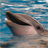# Measure distance between points in a certain order?

4144
4
12-12-2014 03:47 PMNew Contributor II

Hi,

I have points representing animal movement, each point has a date when it was captured in the field. I then created a path of movement (line) showing where the animal moved based on the date order. Now I want to measure the distance btw each date, how far the animal movement from one day to the next. I can't find a tool that will work that I know of.

Any thoughts? Thanks!

4 RepliesbyMVP Esteemed Contributor

Your points much be in a projected coordinate system...(eg UTM etc etc) note just the data frame.  The following field calculator function can be used with:

Parser  Python

Expression  dist_between(!Shape!)

code block below

Ensure that the spacing is correct for the indent levels...ie use 2 spaces per level.  If your data are not projected the answers will be in fractions of degrees and worthless.

```''' input shape field: returns the distance between points and its center
dist_between(!Shape!)    #enter into the expression box'''
x0 = 0.0;  y0 = 0.0
def dist_between(shape):
global x0;  global y0
x = shape.firstpoint.X; y = shape.firstpoint.Y
if x0 == 0.0 and y0 == 0.0:
x0 = x; y0 = y
distance = math.sqrt((x - x0)**2 + (y - y0)**2)
x0 = x;  y0 = y
return distance```New Contributor III

If you already created a line between points. You just need to calculate line distance for each segment by "Calculate Geometry" tool in desired unit.

If you have polyline which is connecting consequence points, then you can split your line by "Split Line at Points" or "Split Line at Vertices" tools. Than you can calculate distance.byEsri Esteemed Contributor

If you use the "Points to Line" tool to create lines from your points, there is an option "Line Field (optional)" that allows you to guide the creation of the lines. In case you have 1 animal and several dates, you can create a date field that will allow the creation of a line per date. If the output is written to a file geodatabase, the output will have a length field with the length of the line (if they are in projected coordinate system as Dan indicated before).

In case you have more than 1 animal, create a field that concatenate the ID of the animal and the date and use that as Line Field. This will create a line per date per animal.New Contributor II

Thank you all, I will try some of those suggestions!